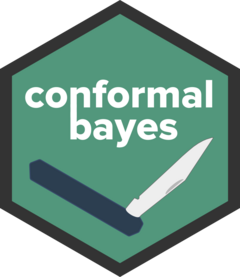# conformalbayesconformalbayes provides functions to construct finite-sample calibrated predictive intervals for Bayesian models, following the approach in Barber et al. (2021). These intervals are calculated efficiently using importance sampling for the leave-one-out residuals. By default, the intervals will also reflect the relative uncertainty in the Bayesian model, using the locally-weighted conformal methods of Lei et al. (2018).

## Installation

You can install the development version of conformalbayes with:

``````# install.packages("devtools")
devtools::install_github("CoryMcCartan/conformalbayes")``````

## Example

``````library(rstanarm)
library(conformalbayes)
data("Loblolly")

fit_idx = sample(nrow(Loblolly), 50)
d_fit = Loblolly[fit_idx, ]
d_test = Loblolly[-fit_idx, ]

# fit a simple linear regression
m = stan_glm(height ~ sqrt(age), data=d_fit,

# prepare conformal predictions
m = loo_conformal(m)

# make predictive intervals
pred_ci = predictive_interval(m, newdata=d_test, prob=0.9)
#>             5%       95%
#> 1  -0.15888597  5.600095
#> 29 25.43314599 30.988491
#> 57 48.67648127 54.182655
#> 2  -0.09561987  5.447242
#> 30 25.42970114 30.938488
#> 72 58.01173186 63.596592

# are we covering?
mean(pred_ci[, "5%"] <= d_test\$height &
d_test\$height <= pred_ci[, "95%"])
#>  0.9117647``````

Read more on the Getting Started page.

Barber, R. F., Candes, E. J., Ramdas, A., & Tibshirani, R. J. (2021). Predictive inference with the jackknife+. The Annals of Statistics, 49(1), 486-507.

Lei, J., G’Sell, M., Rinaldo, A., Tibshirani, R. J., & Wasserman, L. (2018). Distribution-free predictive inference for regression. Journal of the American Statistical Association, 113(523), 1094-1111.